# CFD Simulations AC6-15

(diff) ← Older revision | Latest revision (diff) | Newer revision → (diff)

# CFD Simulations

## Solution Strategy

The computations reported here were performed with the ANSYS-FLUENT (Manual ANSYS Fluent v.17.1) code using the two built-in popular linear eddy-viscosity models (k-ω-SST and realizable k-ε), the Re-stress LRR model (Launder, Reece, & Rodi, 1975) with the linear pressure strain and wall-echo terms, and a DES (based on Menter's k-ω SST model). In parallel, for reference, LES with the WALE subgrid-scale viscosity model (Nicoud & Ducros, 1999) was carried out on finer grids.

The high-Re-number realizable k-ε model and the basic RSM were solved using the standard wall functions. A test with the enhanced wall treatment and the non-equilibrium wall function available in the FLUENT code produced no significant differences in the results. For the k-ω SST model we used the enhanced-wall-treatment ω-equation model (EWT-ω) (ANSYS Fluent v.17.1 Theory Guide).

The computations were performed using the finite-volume method on structured grids. The coupling of the velocity and pressure fields for incompressible flow was ensured using the SIMPLE-C procedure. For the URANS models the convective terms in all equations were discretized by a second-order upwind scheme, whereas the second-order central difference scheme was used for LES and DES (in the LES region). The time derivatives were approximated by an implicit second-order scheme. The time step was set by imposing a CFL<2 constraint. The average CFL number for the 6M grid was 0.35 and for 19.3M grid 0.42. In the bulk of the draft tube the CFL number was almost everywhere less than 1.0 with a maximum of about 1.6 localised in very small areas close to the inlet. Some peaks exceeding the value of 2.0 appeared close to the guide-vane and runner blades, especially their tips, locally reaching values up to 3.0 and even 5.0 for the two respective grids, but caused no numerical instabilities. The statistics were gathered for each run during about 20 sec of real time.

## Computational Domain and Boundary Conditions

The computational domain included all the elements constituting the turbine system (guide vane, runner and draft tube) except for the intake channel (Fig.3). Since the intake channel was not considered in the calculations, the boundary conditions were set at the inlet to the turbine guide vanes by imposing a fixed flowrate measured in the experiment.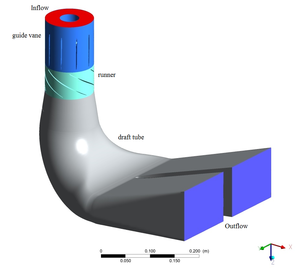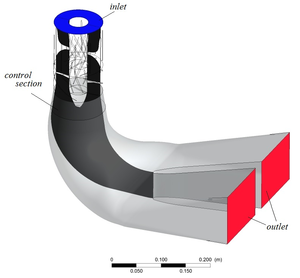Figure 3: Computational domain. Left: outside view of different rig components; right: domain interior displaying the “turbine” model

As the LES and DES require initial time-dependent forcing mimicking turbulence, random velocity fluctuations were imposed at the inlet, generated by the method proposed in Smirnov, Shi, and Celik (2001). The inflow profiles of the mean velocity and turbulence properties at the inlet to the turbine guide vanes were generated by a precursor steady RANS computation (with the k-ε realisable model), of the whole experimental set-up. For the latter, the inflow conditions were evaluated by specifying a uniform velocity, turbulence intensity ${k^{1/2}/U}$of 3%, and the equivalent diameter of the annular passage of 0.2 m, from which the inflow turbulent energy dissipation rate ${\varepsilon }$was evaluated. It is noted that the focus of the present work is on the draft tube for which the entry conditions are generated by the unsteady solutions of flow through the turbine, so that the inflow conditions into the guide vanes are not very influential (Aakti, Amstutz, Casartelli, Romanelli, & Mangani, 2015). The design of the draft tube has two outlets, which is a typical situation for the hydroelectric units operated at the HPP. In the numerical simulation, the conditions of a fixed pressure were set at the outlets.

A specific issue in the computer simulation of hydraulic turbines is the treatment of the rotating runner and the rotor-stator interaction. Several approaches can be found in the literature, i.e. dynamic sliding and moving grid methods and those based on a moving reference frame. The latter is the most common and the simplest way to model the runner rotation and has been used in most of the references cited above, (Zadravec, Basic, & Hribersek, 2007), (Aakti et al., 2015). It assumes that the runner is fixed and the equations are solved in a rotating reference frame. This formulation is often referred to as the "frozen rotor" approach. In this paper, the modelling of the runner rotation was performed in the rotated reference frame for the runner zone. The obtained results are then rotated with the runner rotation speed and as such imposed as the inflow field at the inlet into the draft tube. The earlier test calculations proved that this approach is credible for describing the integral flow characteristics including the dominant flow pulsations (Minakov, Platonov, Dekterev, Sentyabov, & Zakharov, 2015a, 2015b, 2015c). A comparison with the computationally more demanding method using sliding meshes showed that for this type of flows with a focus on draft tube dynamics the results are almost the same.

## Discretisation and Grid Resolution

The computations have been carried out using structured grids with the total number of 2, 6 and 19.3 million (M) cells for the whole domain. The grid was clustered around the runner blades, guide vanes and the draft tube walls (Fig. 4). The average distance of the wall-nearest grid nodes normalized in wall units, y1+, for the 2M grid was 9, for the 6M grid 1.25 and for the 19.3M grid it was 1.05. The corresponding minimum and maximum values are given in Table 1, together with the proportion of the wall surface bounding the computational domain where y1+ exceeds 2.0. As can be seen, for the coarse grid of 2M cells, this portion is 85%, thus disqualifying this grid for the LES. For the 6M grid, the maximum value of y1+ was 23, and the minimum 0.02 with the wall-surface area for which y1+ exceeds 2 being 23% of the entire surface. Likewise, for the 19.3M grid, the maximum y1+ is 4.1, the minimum 0.003. In addition, the wall-area proportion where y1+ exceeds 2.0, is only 8%, confirming a sufficient near-wall resolution.

An impression of the near-wall grid resolution is given in Fig. 5. Interestingly, the LES on a 6M grid produced the main flow features - the pressure pulsations, velocity profiles and the rms of their fluctuations – in good agreement with results for 19.3M cells. This relative insensitivity of LES to the grid refinement above 6M is attributed partially to a carefully designed mesh in the shear layers to meet the resolution criterion based on the “shear” scale (see below), but also to the fact that flow is dominated by large-scale helical vortex structures, unaffected by the small-scale stochastic turbulence that may have remained unresolved on the 6M grid.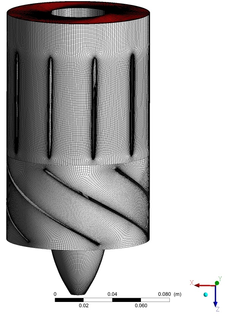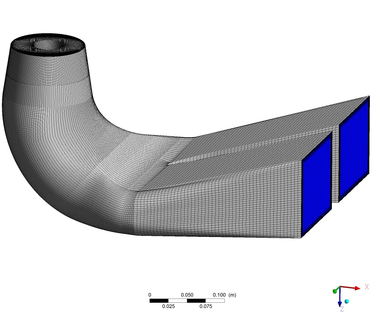Figure 4: Views of the computational grid with 6 million cells in the turbine model (guide vanes and runner) and its draft tube.

 (a) 2 M cells (b) 6 M cells (c) 19.3 M cells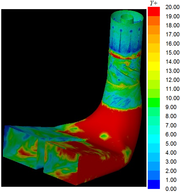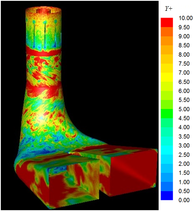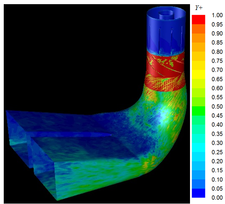Figure 5: Distribution of the wall-nearest grid node y1+on the walls for different meshes. Red colour indicates: (a) y1+ > 20, (b) y1+ > 10, (c) y1+ > 1.

While the 2M grid with y1+ > 20 prevailing over most of the draft tube walls (Fig. 5a) qualifies reasonably well for the wall-function treatment of the wall boundary conditions (used here in the realizable k-ε model and RSM), the use of wall functions on the finer mesh of 6M may cause some concern, since over a good portion of the wall y1+ is smaller (with a maximum of about 23, Table 1). However, as the results show (see below), the less accurate capturing of the velocity in the first few points close to the wall due to a probable overestimation of the wall shear stress, does not seem to affect much the main flow and vortex structures in the bulk of the draft tube. The ANSYS-FLUENT code used here, just as most CFD softwares, provides a remedy for the local areas where y1+ < 11 (the intersection of the “laminar” and the logarithmic velocity profiles). Although such a treatment is not fully justified (as for y1+ < 30 one needs to employ a wall-integration (WIN) scheme that accounts for the viscosity), apart from inadequate reproduction of the wall shear stress, the velocity at few wall-adjacent points is usually reproduced reasonably well. This is confirmed by good agreement for the 6M grid of the RSM calculations despite using the wall functions. Of course, the DES results also agree well with the experiments and observation, but this approach ensures the integration up to the wall and accounts for the viscous effects.

To check further the grid resolution, the characteristic cell size ${\Delta =(\Delta x\times \Delta y\times \Delta z)^{1/3}}$was compared with the Kolmogorov dissipative scale ${L_{K}=(\nu ^{3}/\varepsilon )^{1/4}}$, the energy containing turbulence length scale ${L_{t}=k^{3/2}\varepsilon }$, and the generalized Corrsin “shear” scale ${L_{S}=(\varepsilon /S^{3})^{1/2}}$, where ${\nu }$is the kinematic viscosity, ${k}$is the turbulent kinetic energy, ${\varepsilon }$its dissipation rate (obtained from RSM), and ${S=(S_{ij}S_{ij})^{1/2}}$is the rate-of-strain modulus. This shear scale, characterizing the scale of the turbulence energy production, was found by Picano and Hanjalić (2012) to serve well as a criterion for mesh resolution in free flows: when the grid-cell size was kept smaller than the shear scale (nominally ${\Delta /L_{S}}$< 1.0, and practically ≤ 0.8, relevant primarily in high-shear regions), the LES of a free round jet reproduced well the mean flow and turbulence statistics at very high Re number using a very coarse grid despite the cell sizes being two order of magnitude larger than the Kolmogorov scale. Admittedly, no test is yet available for the near-wall high-shear region, but one can anticipate that a similar criterion would hold. Table 1 summarizes the main parameters for the three grids considered.

 Grid/models y+1,min y+1,max y+aver Wall %y+1 > 2 Δ / LK Δ / LS Δ / Lt 2×106 (RANS, DES) 1.5 110 9.0 85% 6×106 (RANS, LES) 0.02 23 1.25 23% 12 - 20 0.1 - 3.0 0.02 - 0.11 19.3×106 (LES) 0.003 4.1 1.05 8% 4.5 - 10 0.04 - 1.1 0.008 - 0.04

## Experimental verification

The LES reported here were aimed first to establish more comprehensive reference data beyond what is available from the experiment, but also to test the LES grid sensitivity, especially the performance of LES on relatively coarse meshes that do not satisfy the common LES grid resolution criteria, with a view of possible application to real-scale hydromachinery. Surprisingly, the mean velocity profiles obtained with LES on very different grids, 6M and 19.3M shown in Fig. 6 (top) agree very well and also with the experiments. A difference appears in the spectra, but primarily at high wave numbers, which apparently does not affect much the mean flow characteristics, Fig. 6 (bottom). The successful LES reproduction of the main flow features in the flow considered using a relatively coarse mesh of 6M cells can be explained partly by the relatively low Re number of about 29.000 of the scaled-down laboratory draft-tube model, but more by the fact that flow is dominated by the large coherent spiralling vortex (ropes), and thus not much affected by the unresolved small-scale motion. This is further substantiated by a relatively low subgrid-scale viscosity, which reaches a maximum of ${\nu _{sgs}/\nu }$= 14.15 on the 6M grid and 8.32 on the 19.6M grid, with the respective average values of 1.95 and 0.48. Another explanation can be traced to grid optimization made to satisfy the shear-scale criterion, ${\Delta /L_{S}<1}$, congruent with a similar finding for LES of a free round jet reported in Picano and Hanjalić (2012).

 (a) (b)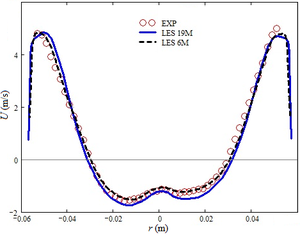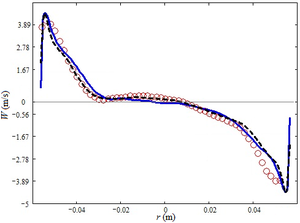(c) (d)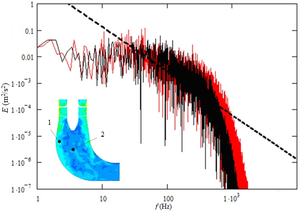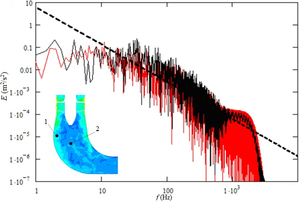Figure 6: Comparison of LES on 6 M and 19.3 M grids for the low part-load operation,${{\mathcal {Q}}/{\mathcal {Q}}_{n}}$=39%. Top: Axial (left) and tangential (right) mean velocity profiles. Bottom: frequency spectra of the turbulent kinetic energy at location 1 (left) and 2 (right); black (dark) 6 M grid, red (gray) 19.3 M grid.

Contributed by: A. Minakov [1,2], D. Platonov [1,2], I. Litvinov , S. Shtork , K. Hanjalić  —

 Institute of Thermophysics SB RAS, Novosibirsk, Russia,

 Siberian Federal University, Krasnoyarsk, Russia,

 Delft University of Technology, Chem. Eng. Dept., Holland.